# Sentiment Analysis by SHAP with Logistic Regression

## Goal¶

This post aims to introduce how to do sentiment analysis using SHAP with logistic regression.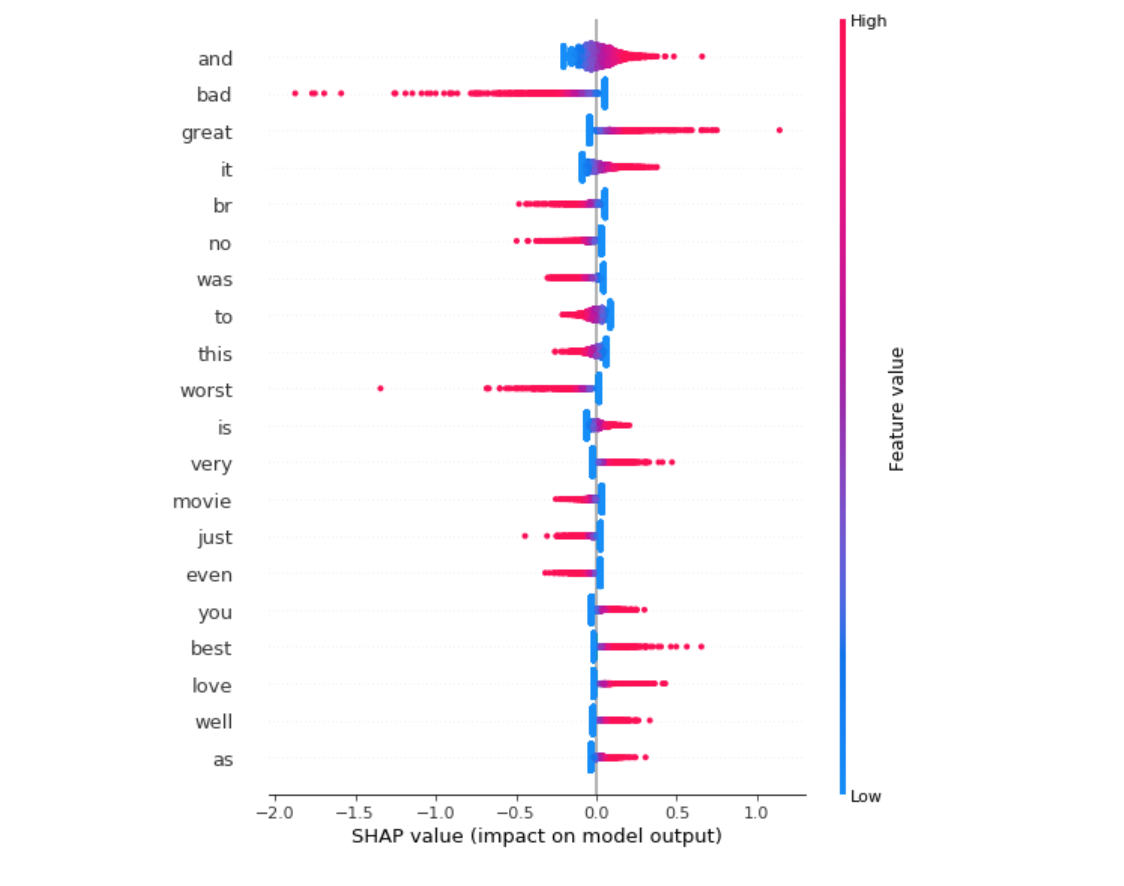Reference

## Libraries¶

In :
import sklearn
from sklearn.feature_extraction.text import TfidfVectorizer
from sklearn.model_selection import train_test_split
import numpy as np
import pandas as pd
import matplotlib.pyplot as plt
import shap
%matplotlib inline

shap.initjs()In :
corpus, y = shap.datasets.imdb()
corpus_train, corpus_test, y_train, y_test = train_test_split(corpus,
y,
test_size=0.2,
random_state=7)


### "Review" example in the corpus¶

In :
# Example of one review
corpus_train[:200]

Out:
"I was excited when I heard they were finally making this horrific event into a movie. The whole era (1980's Southern California) and subject matter (drug and porn industry) is intriguing to me. I thou"
In :
# Target value
y

Out:
False

### Length of each review¶

In :
df_len = pd.DataFrame({'length of each review':[len(c) for c in corpus]})

In :
df_len.hist(bins=100);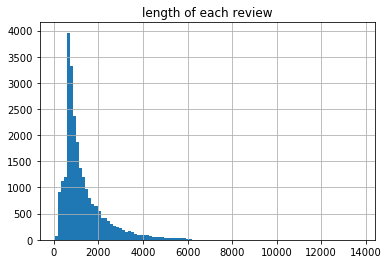In :
pd.Series(y).value_counts().plot(kind='bar', title='Y Label for Corpus');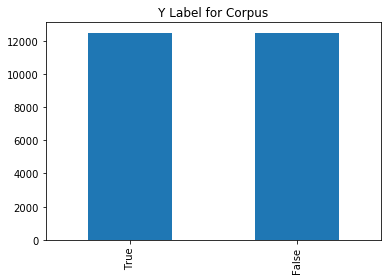### Preprocessing¶

We obtain the apply TFID vectorization to convert a collection of words to a matrix of TF-IDF features.

In :
# Instanciate vectorizer
vectorizer = TfidfVectorizer(min_df=10)

In :
# Train vectorizer
X_train = vectorizer.fit_transform(corpus_train)
# Apply vectorizer to test data
X_test = vectorizer.transform(corpus_test)


### Preprocessed data¶

After applying TFID vecterization, we will obtain the score in a large dimension. In this case, the dimension size is 16416.

In :
X_test.shape

Out:
(1, 16416)
In :
X_test[:5].data[:5]

Out:
array([0.04277508, 0.14533082, 0.02100824, 0.01887938, 0.01996805])

## Train the logistic regression¶

In :
reg = sklearn.linear_model.LogisticRegression(penalty="l2",
C=0.1,
solver='lbfgs')
reg.fit(X_train, y_train)

Out:
LogisticRegression(C=0.1, class_weight=None, dual=False, fit_intercept=True,
intercept_scaling=1, max_iter=100, multi_class='warn',
n_jobs=None, penalty='l2', random_state=None, solver='lbfgs',
tol=0.0001, verbose=0, warm_start=False)

## Sensitivity Analysis¶

To compute SHAP value for the regression, we use LinearExplainer.

### Build an explainer¶

In :
explainer = shap.LinearExplainer(reg,
X_train,
feature_dependence="independent")


### Compute SHAP values for test data¶

In :
shap_values = explainer.shap_values(X_test)
shap_values

Out:
array([[ 6.07763522e-06,  8.53307578e-05, -2.06447665e-06, ...,
-2.60165231e-05, -1.75486507e-05, -2.56592766e-06],
[ 6.07763522e-06,  8.53307578e-05, -2.06447665e-06, ...,
-2.60165231e-05, -1.75486507e-05, -2.56592766e-06],
[ 6.07763522e-06,  8.53307578e-05, -2.06447665e-06, ...,
-2.60165231e-05, -1.75486507e-05, -2.56592766e-06],
...,
[ 6.07763522e-06,  8.53307578e-05, -2.06447665e-06, ...,
-2.60165231e-05, -1.75486507e-05, -2.56592766e-06],
[ 6.07763522e-06,  8.53307578e-05, -2.06447665e-06, ...,
-2.60165231e-05, -1.75486507e-05, -2.56592766e-06],
[ 6.07763522e-06,  8.53307578e-05, -2.06447665e-06, ...,
-2.60165231e-05, -1.75486507e-05, -2.56592766e-06]])

### Plot the features importance¶

In :
X_test_array = X_test.toarray()
shap.summary_plot(shap_values,
X_test_array,
feature_names=vectorizer.get_feature_names())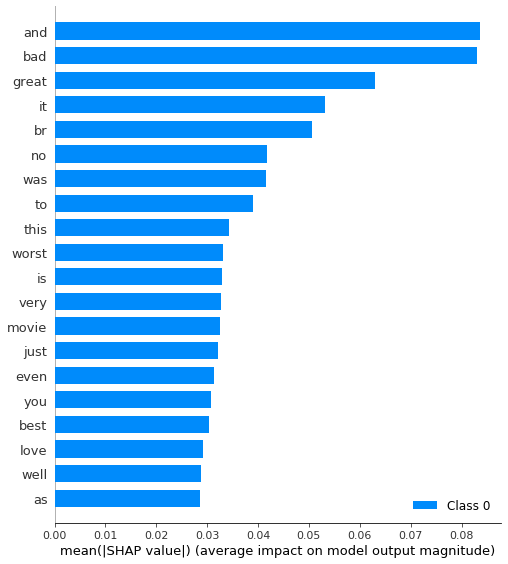### Plot the SHAP values for top features¶

In :
# shap_values does not work since it is recognized as list and default to bar chart only.
# so it changed to shap_values
shap.summary_plot(shap_values,
X_test_array,
feature_names=vectorizer.get_feature_names(),
plot_type='dot')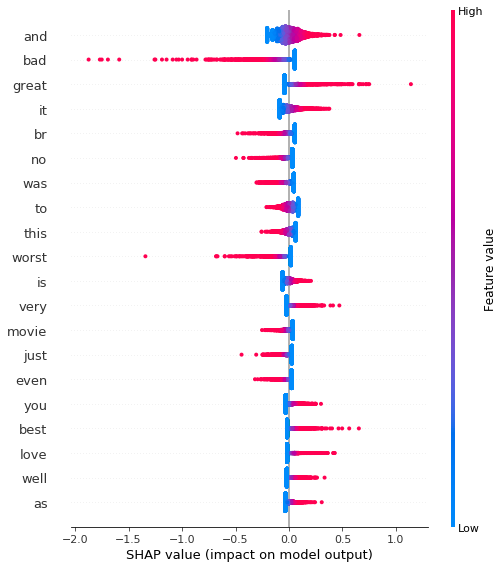### Explain the sentiment for one review¶

I tried to follow the example notebook Github - SHAP: Sentiment Analysis with Logistic Regression but it seems it does not work as it is due to json seriarization.

In :
X_test_array[i, :]

Out:
array([0., 0., 0., ..., 0., 0., 0.])
In :
ind = 0
shap.force_plot(
explainer.expected_value, shap_values[ind,:], X_test_array[ind,:],
feature_names=vectorizer.get_feature_names()
)

---------------------------------------------------------------------------
TypeError                                 Traceback (most recent call last)
<ipython-input-100-0ae897f509cf> in <module>
2 shap.force_plot(
3     explainer.expected_value, shap_values[ind,:], X_test_array[ind,:],
----> 4     feature_names=vectorizer.get_feature_names()
5 )

~/anaconda3/envs/py367/lib/python3.6/site-packages/shap/plots/force.py in force_plot(base_value, shap_values, features, feature_names, out_names, link, plot_cmap, matplotlib, show, figsize, ordering_keys, ordering_keys_time_format, text_rotation)
132         )
133
--> 134         return visualize(e, plot_cmap, matplotlib, figsize=figsize, show=show, text_rotation=text_rotation)
135
136     else:

~/anaconda3/envs/py367/lib/python3.6/site-packages/shap/plots/force.py in visualize(e, plot_cmap, matplotlib, figsize, show, ordering_keys, ordering_keys_time_format, text_rotation)
271             return AdditiveForceVisualizer(e, plot_cmap=plot_cmap).matplotlib(figsize=figsize, show=show, text_rotation=text_rotation)
272         else:
274     elif isinstance(e, Explanation):
275         if matplotlib:

~/anaconda3/envs/py367/lib/python3.6/site-packages/shap/plots/force.py in html(self, label_margin)
362     document.getElementById('{id}')
363   );
--> 364 </script>""".format(err_msg=err_msg, data=json.dumps(self.data), id=id_generator()))
365
366     def matplotlib(self, figsize, show, text_rotation):

~/anaconda3/envs/py367/lib/python3.6/json/__init__.py in dumps(obj, skipkeys, ensure_ascii, check_circular, allow_nan, cls, indent, separators, default, sort_keys, **kw)
229         cls is None and indent is None and separators is None and
230         default is None and not sort_keys and not kw):
--> 231         return _default_encoder.encode(obj)
232     if cls is None:
233         cls = JSONEncoder

~/anaconda3/envs/py367/lib/python3.6/json/encoder.py in encode(self, o)
197         # exceptions aren't as detailed.  The list call should be roughly
198         # equivalent to the PySequence_Fast that ''.join() would do.
--> 199         chunks = self.iterencode(o, _one_shot=True)
200         if not isinstance(chunks, (list, tuple)):
201             chunks = list(chunks)

~/anaconda3/envs/py367/lib/python3.6/json/encoder.py in iterencode(self, o, _one_shot)
255                 self.key_separator, self.item_separator, self.sort_keys,
256                 self.skipkeys, _one_shot)
--> 257         return _iterencode(o, 0)
258
259 def _make_iterencode(markers, _default, _encoder, _indent, _floatstr,

~/anaconda3/envs/py367/lib/python3.6/json/encoder.py in default(self, o)
178         """
179         raise TypeError("Object of type '%s' is not JSON serializable" %
--> 180                         o.__class__.__name__)
181
182     def encode(self, o):

TypeError: Object of type 'matrix' is not JSON serializable